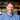January 30, 2019

# Rotating Arrays: Two Ways

How would you rotate an array’s elements if you were given an array and a number of left rotations?

(A left rotation is defined as moving the first element to the last position of the array and shifting all subsequent elements one place to the left.

This post explores two separate approaches and was inspired by a HackerRank challenge.1

Example: `leftRotate([1,2,3,4,5],2) // [3,4,5,1,2]` by way of the following steps: ` [2,3,4,5,1] -`

There are a few potential gotchas in how to approach this problem. The biggest one to me was to realize that `d` can be less than or greater than the total length of the array.

With that as the case, I wanted to understand what the effective (i.e. the total number of steps that actually needed to happen) number of rotations would be.

For example, if we did `leftRotate([1,2,3,4],6)` We would have:

``````0) [1,2,3,4] ->
1) [2,3,4,1] ->
2) [3,4,1,2] ->
3) [4,1,2,3] ->
4) [1,2,3,4] ->
5) [2,3,4,1] ->
6) [3,4,1,2]``````

But steps 1 and 5, 2 and 6 are the same! That’s because `6 % 4 = 2`, so the effective number of rotations is actually only two.

Knowing this, we can reduce the number of steps dramatically. In our small example we had a 66% decline in the number of steps.

Just imagine if the number of steps and array size diverge even further!

The iterative approach

``````/**
* Left Rotation
* @args {Array} a - An array of integers
* @args {number} d - The number of left-shift rotations to perform
* @returns {string} Space separated integers
* @example <caption> Examples of rotLeft </caption>
* rotLeft([1,2,3,4], 6) // returns [3,4,1,2]
* rotLeft([1,2,3,4], 3) // returns [4,1,2,3]
*/
function rotLeft(a, d) {
//calculate effective rotation (d % a.length)
let effectiveRotation = d % a.length

// rotate the elements of a
for (let i = 0; i < effectiveRotation; i += 1) {
a = a.slice(1).concat(a)
}
return a
}``````

# A Loop-less Approach

Instead of using loops, we can also go directly to the point in the array to create new sub-arrays.

However, since arrays are single blocks of memory, the creation of two new sub-arrays and their subsequent concatenation into the new final array would still be linear time due to the time it takes to construct the arrays.

``````/**
* Left Rotation
* @args {Array} a - An array of integers
* @args {number} d - The number of left-shift rotations to perform
* @returns {string} Space separated integers
* @example <caption> Examples of rotLeft </caption>
* rotLeft([1,2,3,4], 6) // returns [3,4,1,2]
* rotLeft([1,2,3,4], 3) // returns [4,1,2,3]
*/
function rotLeft(a, d) {
//calculate effective rotation (d % a.length)
let effectiveRotation = d % a.length

// split a at index of effective rotation into left and right
let leftPortion = a.slice(0, effectiveRotation) // O(n)
let rightPortion = a.slice(effectiveRotation) // O(n)

// concat left to right
return rightPortion.concat(leftPortion) // O(n)
}``````

# Conclusion

Though it’s still O(n), the loop-less approach is almost always going to be more efficient than the iterative approach.

Though they both have a time complexity of O(n). The loop-less approach has three methods that are all O(n), and as such is ~3n.

Meanwhile, the iterative approach is O(effectiveRotations * n) because each element in the array has to shift to the left for each effective iteration.

This is one of the drawbacks of arrays as data structures. Access is fast, but insertion / deletion is not (except at the end and assuming there’s sufficient space).

## FootnotesThanks for reading! My name's Stephen Weiss. I live in Chicago with my wife, Kate, and dog, Finn.